Monday , May 27 2019
Recent Post
Home / Quantitative Aptitude Tricks / Compound Interest Tricks & Tips

# Compound Interest Tricks & TipsNABARD Grade A & B Online Course Get Course Now 150+ RRB NTPC Previous Solved Papers PDF Free Download NowRBI Grade B Complete Online Course Register Today RRB JE Free Study Material PDF Books Free Download Now RRB NTPC Free Study Material PDF Free Download NowAll Exam Free Study Group (Free Videos,Pdf,Mock etc) Join Right Now

# Compound Interest Tricks & Tips

Compound Interest :- Sometimes it so happens that the borrower and the lender agree to fix up a certain unit of time, say yearly or half-yearly or quarterly to settle the precious account.

In such cases, the amount after first unit of times becomes the principal for the second unit the amount after second unit becomes the principle for the third unit and so on.

After a specified period, the difference between the amount and the money borrowed is called the Compound Interest (abbreviated as C.I.) for that period.

###Register For NABARD Grade A & B CourseClick Here

####Complete Maths Shortcut eBook > Click To Buy @99rs Only

Compound Interest is the interest calculated on the initial principal and the accumulated interest of previous periods of a deposit or loan.

In easy words, it can be said as “interest on interest”. It makes a deposit or loan grow faster as compared to simple interest. The interest at which compound interest accumulates depends on the frequency of compounding; more the number of compounding periods, the greater the compound interest.

Note: The interest for the first month is same in both Simple Interest and Compound Interest. From second month, the interest starts changing.

The formula for Compound Amount

P [1+ R/100]n [When money is compounded annually] = P [1+ R/(2*100)]2n [When money is compounded half-yearly] = P [1+ R/(12*100)]12n [When money is compounded monthly] Also, A = CI + P
Where,
P= Principal
R= Rate of Interest
n=Time (in years)
A= Amount
CI= Compound Interest

Note: The above formula: A = CI + P will give us total amount. To get the Compound Interest only, we need to subtract the Principal from the Amount.

The table given below lists the values of an initial investment, P = Re. 1 for certain time periods and rates of interest, calculated at both, simple and compound interest. If memorized this would be of great help in time management during the exam,

Rate⇒
Time⇓
5% 10% 15% 20%
SI CI Diff SI CI Diff SI CI Diff SI CI Diff
1 year 1.05 1.05 0 1.1 1.1 0 1.2 1.2 0 1.2 1.2 0
2 years 1.10 1.1025 0.0025 1.2 1.21 0.01 1.4 1.44 0.04 1.6 1.69 0.09
3 years 1.15 1.1575 0.0075 1.3 1.331 0.031 1.6 1.728 0.128 1.9 2.197 0.297

To understand the above discussed concepts, let’s try some questions.

Solved Questions

Questions 1:Find the amount if Rs 20000 is invested at 10% p.a. for 3 years.

Solution: Using the formula:A= P [1+ R/100]n
A = 20000 [1 + (10/100)]3
On Solving, we get A = Rs. 26620

Question 2: Find the CI, if Rs 1000 was invested for 1.5 years at 20% p.a. compounded half yearly.

Solution: As it is said that the interest is compounded half yearly. So, the rate of interest will be halved and time will be doubled.

CI = P [1+(R/100)]n – P
CI = 1000 [1+(10/100)]3– 1000
On Solving, we get
CI = Rs. 331

Question 3: The CI on a sum of Rs 625 in 2 years is Rs 51. Find the rate of interest.

Solution: We know that A = CI + P
A = 625 + 51 = 676
Now going by the formula: A = P [1+(R/100)]n
676 = 625 [1+(R/100)]2
676/625 = [1+(R/100)]2
We can see that 676 is the square of 26 and 625 is the square of 25
Therefore, (26/25)2 = [1+(R/100)]2
26/25 = [1+(R/100)] 26/25 – 1 = R/100
On solving, R = 4%

Question 4:A sum of money is put on CI for 2 years at 20%. It would fetch Rs 482 more if the interest is payable half yearly than if it were payable yearly. Find the sum.

Solution: Let the Principal = Rs 100
When compounded annually,
A = 100 [1+20/100]2
When compounded half yearly,
A = 100[1+10/100]4
Difference, 146.41 – 144 = 2.41
If difference is 2.41, then Principal = Rs 100
If difference is 482, then Principal = 100/2.41 × 482
P = Rs 20000.

Question 5: Manish invested a sum of money at CI. It amounted to Rs 2420 in 2 years and Rs 2662 in 3 years. Find the rate percent per annum.

Solution: Last year interest = 2662 – 2420 = Rs 242
Therefore, Rate% = (242 * 100)/(2420 * 1)
R% = 10%
Important Formula: To find the difference between SI and CI for 2 years, we use the formula Difference = P[R/100]2

Question 6:The difference between SI and CI for 2 years @ 20% per annum is Rs 8. What is the principal?

Solution: Using the formula: Difference = P (R/100)2
8 = P[20/100]2
On Solving, P = Rs 200

We all know the traditional formula to compute compound interest.

CI = P*(1+R/100)^N – P

This calculation gets very tedious when N>2 (more than 2 years). The method suggested below is a very simple way to get CI/Amount after ‘N’ years.

You need to recall the old Pascal’s Triangle in following way:

Code:

Number of Years (N)

——————-

1                        1

2                    1   2   1

3                  1   3   3   1

4                1   4   6   4   1

.              1 …. …. … … …. …   1

Example: P = 1000, R=10 %, and N=3 years. What is CI & Amount?

Step 1: 10% of 1000 = 100, Again 10% of 100 = 10 and 10% of 10 = 1

We did this three times becoz N=3.

Step 2:

Now Amount after 3 years = 1 * 1000 + 3 * 100 + 3 * 10 + 1 * 1 = Rs.1331/-

The co-efficients – 1,3,3,1 are lifted from the pascal’s triangle above.

Step 3:

CI after 3 years = 3*100 + 3*10 + 3*1 = Rs.331/- (leaving out first term in step 2)

If N =2, we would have had, Amt = 1 * 1000 + 2 * 100 + 1 * 10 = Rs. 1210/-

CI = 2 * 100 + 1* 10 = Rs. 210/-

This method is extendable for any ‘N’ and it avoids calculations involving higher powers on ‘N’ altogether!

A variant to this short cut can be applied to find depreciating value of some property. (Example, A property worth 100,000 depreciates by 10% every year, find its value after ‘N’ years).

N.B Prefer this method if N>=3. It will always work and it will save your time like anything.

## Shortcuts for Calculating Compound Interest

Here is a shortcut trick for calculation of compound interest for 2 and 3 years. Instead of putting formulas and doing long calculation you can use the short tricks according to given percentage

CI for 2 Years                                                                                   CI For 3 Years

 2% 4.04% 2% 6.12% 3% 6.09% 3% 9.27 4% 8.16% 4% 12.48 5% 10.25% 5% 15.76 6% 12.36% 6% 19.10 7% 14.49& 7% 22.50 8% 16.64% 8% 25.97 9% 18.81% 9% 29.50 10% 21% 10% 33.10 11% 23.21% 11% 36.76 12% 25.44% 12% 40.49 13% 27.69 13% 44.28 14% 29.96 14% 48.15 15% 32.25 15% 52.08 16% 34.56 16% 56.08 17% 36.89 17% 60.16 18% 39.24 18% 64.30 19% 41.61 19% 68.51 20% 44.00 20% 72.80

Some important formula of Compound Interest

• A = Amount.
P = Principal.
R = Rate of Interest.
N = Number of Years.

• Type I : Interest compounded yearly :
A = P ( 1 + r / 100 )n

• Type II : Interest compounded half – yearly :
Amount = P [ 1 + r / 2 / 100 ]4n or = P = [ 1 + r / 200 ] 2n

• Type III : Interest compounded quarterly :
Amount = P [ 1 + r / 4 / 100 ] or = P [ 1 + r / 400 ] 4n

In Compound Interest problems asked in exams up to the period of 3 years.
In case we apply basic formula: Amount =Principal ( 1 + r / 100 )n here r = Rate and n = Time
As consider if Principal is Rs. 1, then the it will be in first year and second and third years.
( 1 + r / 100 )1
( 1 + r / 100 )2
( 1 + r / 100 )3
If the rate of interest is 3%, then the value will be …….
In first year = (23 / 21 ) = 23 / 21.
In second year = ( 23 / 21 )2 = 529 / 441.
In Third year = ( 23 / 21 )3 = 12167 / 9261.

Compound Interest Tricks: Results related to Multiple Compounding in a year:

In each of the following results, we use the following denotations:
A = future value
P = principal amount (initial investment)
r = annual nominal interest rate
n = number of times the interest is compounded per year
t = number of years for which the money is borrowed

Compound Interest Trick 1: If interest is not compounded yearly then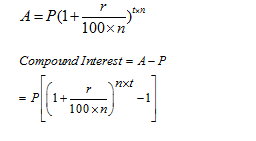where

n= number of times compounding is done
if compounding is done half yearly then n = 2
if compounding is done quarterly  then n = 4
when compounded monthly then n=12

Amount for Half Yearly Compounding, A = P {1+(R/2)/ 100}2T
(compound interest applied two times in a year)
Like Half Yearly Compound Interest, we can calculate the amount for Quarterly Compounding.
Amount for Quarterly Compounding,A = P {1+(R/4)/ 100}4T

Example 1: Sona deposited Rs. 4000 in a bank for 2 years at 5% p.a.rate. Find the amount received by her from the bank if interest is compounded half yearly.

Solution:
Principal value = Rs. 4000
Rate = 5%
Time = 2 years
Since the interest is compounded half yearly so 2 years = 4 times in two years
So, we have
A = P {1+(R/2)/ 100}2T
A= 4000{1+ (5/2)/100}4
A = 4000 x 41/40 x 41/40 x 41/40 x 41/40
A = Rs. 4415.25
So, Sona received Rs. 4415.25 from the bank after two years

Example 2: Manpreet lent Rs 5000 to Richa at 10% rate for 1 year. But she told her that she will take her money on compound interest. Find the amount of interest received by Manpreet when compounded quarterly.

Solution:
Principal value = Rs. 5000
Rate = 10%
Time = 1 year
The interest is compounded quarterly, that is 4 times in 1 year.
We use the formula=A = P {1+(R/4)/ 100}4T
A= 5000{1+ (10/4)/100}4
A = 5000 x 41/40 x 41/40 x 41/40 x 41/40
A = Rs. 5519.064
So, Manpreet received Rs. 5519.064 from Richa and the total amount of interest received by her is 5519.064 – 5000 = Rs. 519.06

Compound Interest Tricks: When the rate % is not same for every year and interest is taken on yearly basis then amount can be calculated as:Example 3:  Find the compound interest on 16000 in 2 years , while rate of interest at first year is 3% and for the second year is 4%.

Solution: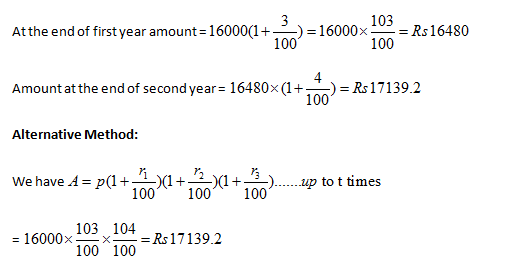Compound Interest Tricks: When interest is compounded yearly and time is given in fraction

If time is given in mixed fraction

Use formula,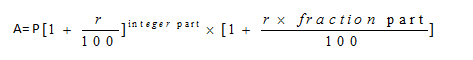Example 4: The compound interest on Rs. 8,000 at 15% per annum for 2 years 4 months, compounded annually is:

Solution: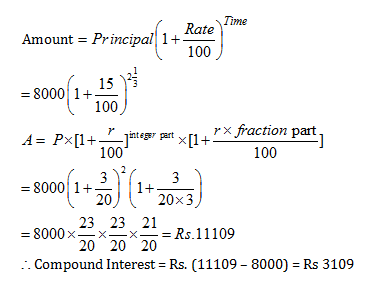### Compound Interest Tricks: Practice Questions

Question 1: A certain sum, invested at 4% per annum compound interest, compounded half – yearly, amounts to Rs 7,803 at the end of one year. The sum is

A. Rs 7,000
B. Rs 7,200
C. Rs 7,500
D. Rs 7,700

### Answer and Explanation

Option CLet the sum be Rs. P.

As, the interest is compounded half-yearly.

R = 2%, T = 2 half years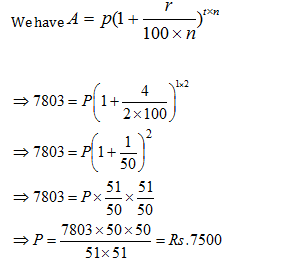Question 2: If the rate of interest be 4% per annum for first year, 5% per an­num for second year and 6% per annum for third year, then the compound interest of Rs. 10,000 for 3 years will be

A. Rs. 1,600
B. Rs. 1,625.80
C. Rs. 1,575.20
D. Rs. 2,000

### Answers and Explanations

Option CWhen the rate of interest is different for the different years, we can use the following formula to find the amount.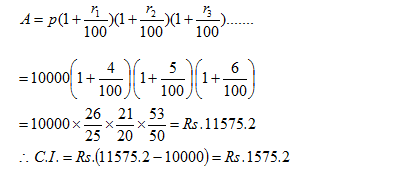Question 3: The compound interest on Rs. 16,000 for 9 months at 20% per annum, interest being com­pounded quarterly, is

A. Rs. 2,520
B. Rs. 2,524
C. Rs. 2,522
D. Rs. 2,518

### Answers and Explanation

Option CThe interest is compounded quarterly. SoQuestion 4: The compound interest on Rs. 6,000 at 10% per annum for 3/2 years, when the interest be¬ing compounded annually, is
A. Rs. 930
B. Rs. 870
C. Rs. 910
D. Rs. 900

### Answers and Explanations

Option AIt is best to be selective with these compound interest shortcuts and use them to the best of your ability.

In each of the following results, we use the following denotations:

A = future value
P = principal amount (initial investment)
r = annual nominal interest rate
n = number of times the interest is compounded per year
t = number of years for which the money is borrowed

Let’s get started and cover some of these shortcuts.

Compound Interest Shortcuts: Tooltip-1

A sum of money placed at compound interest becomes x time in ‘a’ years and y times in ‘b’ years. These two sums can be related by the following formula:Derivation for this result:
We use the basic formula for calculating Compound Interest:For condition 1, a sum of money becomes x times in “a” years.
Therefore, using the formula for calculating Compound Interest: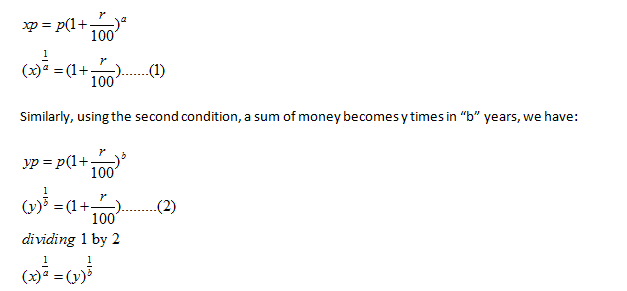Example-1: A sum of money placed at compound interest doubles itself in 4 years.In how many years will it amount to 16 times itself?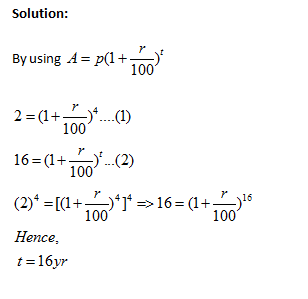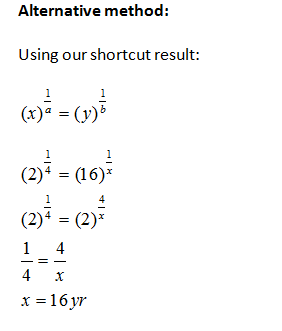Compound Interest Shortcuts: Tooltip 2

If an amount of money grows up to Rs x in t years and up to Rs y in (t+1) years on compound interest, then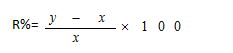Derivation for this result:

Principal + CI for t years = x ……   (1)
Principal + CI for (t+1) years= y  …….  (2)
(2) – (1) =>CI for last year = y-x
Which is basically the simple interest upon xExample-2: An amount of money grows upto Rs 3000 in 3 years and upto Rs 4000 in 4 years on compound interest. What will be the rate percent?

Solution:
Principal + CI for 3 years = 3000 ……   (1)
Principal + CI for 4 years= 4000  …….  (2)
Hence (2) – (1) =>CI for 4th year = 4000-3000= Rs 1000
Which is basically the simple interest upon 3000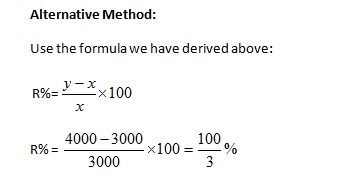Compound Interest Shortcuts: Tooltip 3

A sum at a rate of interest compounded yearly becomes Rs. A1 in n years and Rs. A2 in (n + 1) years, then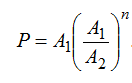Example-3: A sum of money invested at compound interest amounts to Rs. 100 at the end of first year and Rs. 120 at the end of second year. The sum of money is :

Solution:
Simple Interest for one year = compound interest for one year
Interest on Rs. 100 for 1 year = 120-100= Rs. 20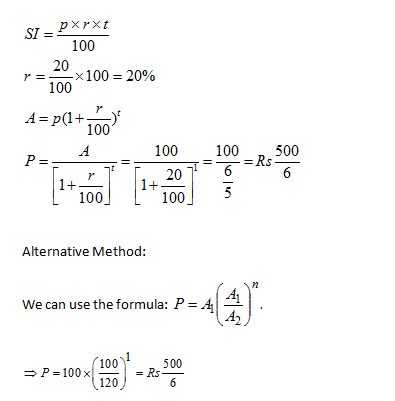Compound Interest Shortcut: Tooltip 4

If a certain sum becomes x times of itself in t years, the rate of compound interest will be equal toDerivation for this result:

Use the formula for Compound Interest Calculation:Sum becomes x times of itself in t years soExample 4:  If a certain sum becomes 16 times in 2 years ,what will be the rate of compound interest?

Solution:

Using the formula derived above:Compound Interest Shortcut: Tooltip 5If the compound interest on a certain sum for 2 years is CI and simple interest for two years is SI ,then rate of interest per annum  is

If the compound interest on a certain sum for 2 years is CI and simple interest for two years is SI ,then rate of interest per annum  is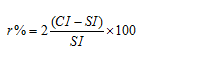Derivation for this result: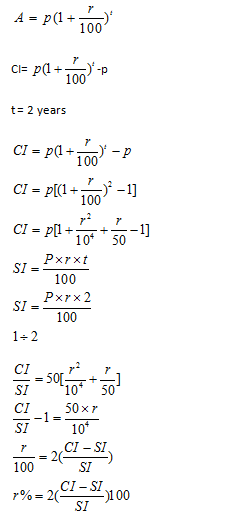Example 5: If the compound interest on a certain sum for 2 years is 20rs and simple interest for two years is 10rs  ,then what wil be the  rate of interest per annum  ?

Solution:

Using the formula derived above:### Compound Interest Solved Problems using Compound Interest Shortcuts:

Let’s go through some compound interest solved problems and learn how to use and implement compound interest shortcuts in actual problem solving. Remember, till the time you actually solve questions using these tricks, you won’t be able to memorize and understand them. Go through compound interest solved problems and hone your skills for the topic.

Question 1: What sum of money at com­pound interest will amount to Rs. 650 at the end of the first year and Rs. 676 at the end of the second year?

A. Rs. 600
B. Rs. 600.25
C. Rs. 625
D. Rs. 625.25

### Answers and Explanations

Option CUsing the formula we have derived in the article for this exercise:

If an amount of money grows up to Rs x in t years and up to Rs y in (t+1) years on compound interest, thenQuestion 2: If the amount is 2.25 times of the sum after 2 years at com­pound interest (compound annu­ally) , the rate of interest per an­num is :

A. 25%
B. 30%
C. 45%
D. 50%

### Answers and Explanations

Option DUsing the formula we have derived in the article for this exercise:

If a certain sum becomes x times of itself in t years, the rate of compound interest will be equal toQuestion 3: A sum of money doubles itself in 4 years at compound interest. It will amount to 8 times itself at the same rate of interest in:

A. 18 years
B. 12 years
C. 16 years
D. 24 years

### Answes and Explanations

Option BUsing the formula we have derived in the article for this exercise:

A sum of money placed at compound interest becomes x time in ‘a’ years and y times in ‘b’ years. These two sums can be related by the following formula:Question 4: A sum borrowed under com­pound interest doubles itself in 10 years. When will it become fourfold of itself at the same rate of interest?

A. 15 years
B. 20 years
C. 24 years
D. 40 years

### Answers and Explanations

Option BUsing the formula we have derived in the article for this exercise:

A sum of money placed at compound interest becomes x time in ‘a’ years and y times in ‘b’ years. These two sums can be related by the following formula:Question 5: A sum of money becomes eight times of itself in 3 years at com­pound interest. The rate of interest per annum is

A. 100%
B. 80%
C. 20%
D. 10%

### Answers and Explanations

Option ALet the principal be Rs. x and the rate of compound interest be r% per annum.

Then,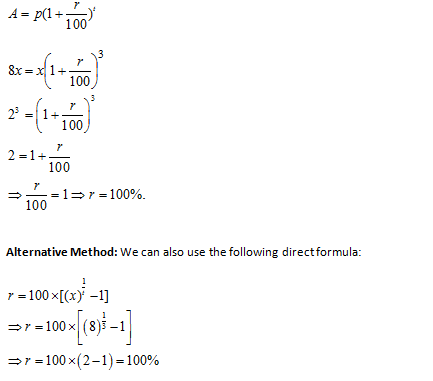#### Important Facts & Formulas on Compound Interest

Case 1: Let principle = P, time = n years and rate = r% per annum and let A be the total amount at the end of n years, thenExample: Albert invested an amount of Rs.8000 in a fixed deposit scheme for 2 years at compound interest rate 5 p.c.p.a. how much amount will Albert get on maturity of the fixed deposit.

Solution:

Amount = Rs.= 8820 Rs.

Case 2: When compound interest is reckoned half-yearly.

If the annual rate is r% per annum and is to be calculated for n years, then in this case, rate = (n/2%) half-yearly and time = (2n) half-yearly.

Form the above we getExample: Sam investment Rs.15,000 @ 10% per annum for one year. If the interest is compounded half-yearly, then the amount received by Sam at the end of the year will be.

Solution:

P = Rs. 15000; R = 10% p.a = 5% half-year, T = 1 year = 2 half year

Amount = Rs= Rs.16537.50

Case 3: When compound interest is reckoned quarterly.

In this case, rate = (r/4%) quarterly and time = (4n) quarter years.

As before,Example:

Find the compound interest on Rs. 15,625 for 9 months at 16% per annum compounded quarterly.

Solution:

P = Rs. 15625, n= 9 months = 3 quarters, R = 16% p.a. = 4% per quarter.

Amount = Rs.= Rs.17576 —> C.I = Rs. (17576 – 15625 ) = Rs. 1951.

Note: The difference between the compound interest and the simple interest over two years is given by

{(Pr^2)/100^2}  or  {P (R/100)^2}

Case 4: When interest is compounded annually but time is in fraction, say  years.

Amount =Example:

What is the difference between the compound interest on Rs. 5000 for  at 4% per annum compounded yearly and half-yearly?

Solutions:

C.I. when interest is compounded yearly .= Rs.5304

C.I. when interest is compounded half-yearlyDifference = Rs.(5306.04 – 5304 ) = Rs.2.04.

Case 5: when rates are different for different years, say  for 1st, 2nd, 3rdyear respectively.

Then, amount =Example:

The population of Jhumri Tilaiya increases by 10% in the first year, it increase by 20% in the second year and due to mass exodus, it decreases by 5% in the third year. What will be its population after 3 years, if today it is 200,000?

Solution:

Population at the end of 1 year will be 10,000 + 10% of 10,000= 11,000

At the end of second year it will be 11,000 + 20% of 11,000 = 13,200

At the end of third year it will be 13,200 – 5% of 13, 20  = 12,540

Case 6: Present worth of Rs.x due n years hence is given by:

Present Worth = [x/{1+(r/100)^n}]

Example:

The principle that amounts to Rs.4913 in 3 years at  per annum compound interest compounded annually, is :

Solution:

Principle = Rs.# Practice Sets for Compound Interest

• The compound interest on a certain sum for 2 years is Rs. 786 and S.I. is Rs. 750. If the sum is invested such that the S.I. is Rs. 1296 and the number of years is equal to the rate per cent per annum, Find the rate of interest?
A.4%
B.5%
C.6%
D.8%
E.2%
Answer & Explanation
Answer – C.6%
Explanation :
CI for 2 years = Rs. 786
SI for 2 years = Rs. 750
36/360 * 100 = 10%
P for first year = 3600
P*x*x/100 = 1296
x = 6%
• Hari took an educational loan from a nationalized bank for his 2 years course of MBA. He took the loan of Rs.5 lakh such that he would be charged at 7% p.a. at CI during his course and at 9% CI after the completion of the course. He returned half of the amount which he had to be paid on the completion of his studies and remaining after 2 years. What is the total amount returned by Hari?
A.Rs. 626255
B.Rs. 626277
C.Rs. 616266
D.Rs. 626288
E.None of these
Answer & Explanation
Answer – D.Rs. 626288
Explanation :
5,00,000 * (1.07)² = 572450
Returned amount = 286225
After two years = 286225 * (1.09)² = 340063
Total amount = 286225 + 340063 = 626288
• Rs.20,000 was invested by Mahesh in a FD @ 10% pa at CI. However every year he has to pay 20% tax on the CI. How much money does Mahesh have after 3 years?
A. 25694
B. 25594
C. 25394
D. 25194
E.None of these
Answer & Explanation
Answer – D. 25194
Explanation :
(20000*(1.08)³)=25194
• Leela takes a loan of Rs. 8400 at 10% p.a. compounded annually which is to be repaid in two equal annual installments. One at the end of one year and the other at the end of the second year. The value of each installment is?
A. 4200
B. 4140
C. 4840
D. 5640
E. None of these
Answer & Explanation
Answer – C. 4840
Explanation :
8400 = x*(210/121) => 4840
• A sum of money lent at compound interest for 2 years at 20% per annum would fetch Rs.723 more, if the interest was payable half yearly than if it was payable annually. The sum is ____
A.Rs. 20000
B.Rs. 15000
C.Rs. 30000
D.Rs. 45000
E.None of these
Answer & Explanation
Answer – C.Rs. 30000
Explanation :
sum – Rs.x
C.I. compounded half yearly = (4641/10000)x
C.I. compounded annually = (11/25)x
(4641/10000)x – (11/25)x = 723
x = 30000
• A sum of Rs.7140 is to be divided between Anita and Bala who are respectively 18 and 19 yr old, in such a way that if their shares will be invested at 4% per annum at compound interest, they will receive equal amounts on attaining the age of 21 year. The present share of Anita is
A. 4225
B. 4352
C. 3500
D. 4000
E. None of these
Answer & Explanation
Answer – C. 3500
Explanation :
Amount got by Anita after 3 yr = Amount got by Bala after 2 yr
x*(26/25)³ = (7140 – x)*(26/25)
26/25 = 7140 – x / x
x = 3500
• Suresh borrows Rs.6375 to be paid back with compound interest at the rate of 4 % pa by the end of 2 year in two equal yearly installments. How much will each installment will be?
A.3840
B.3380
C.4800
D.Data inadequate
E.None of these
Answer & Explanation
Answer – B.3380
Explanation :
25x/26 + 625/676x  = 6375
x = (6375 * 676)/1275 = 3380
• A sum of Rs. 8400 was taken as loan. This is to be paid in two equal annual installments. If the rate of interest be 20% compounded annually, then the value of each installment is
A. 5400
B. 5700
C. 5100
D. 5200
E. None of these
Answer & Explanation
Answer – A. 5400
Explanation :
Let value of each installment be X.
X/(1 + 20/100) + X/(1 + 20/100)² = 8400
⇒ X(5/6 + 25/36) = 8400
⇒ X(56/36) = 8400
X = 5400
• During the first year the population of a village is increased by 5% and the second year it is diminished by 5%. At the end of the second year its population was 31500. What was the population at the beginning of the first year?
A. 35500
B. 31578
C. 33500
D. 33000
E. None of these
Answer & Explanation
Answer – B. 31578
Explanation :
x * 105/100 * 95/100 = 31500
x = 31500 * 100/105 * 100/95
D = 31578
• If Rs. 7200 amounts to Rs.10368 at compound interest in a certain time , then Rs. 7200 amounts to what in half of the time?
A. 8640
B. 8600
C. 8800
D. 8520
E. None of these
Answer & Explanation
Answer – A. 8640
Explanation :
Let rate = R% and time = n year
Then, 10368 =7200(1+R/100)n
⇒ (1+R/100)n = 10368/7200 = 1.44
∴ (1 + R/100)n/2 = √1.44 = 1.2
∴ Required amount for n/2 yr
= 7200(1+ R/100)n/2
= 7200 x 1.2 = Rs. 8640

• A part of 70000 is lent out at 10% annum. The rest of the amount is lent out at 5% per annum after one year. The ratio of interest after 3 years from the time when first amount was lent out is 1:2. Find the second part that was lent out at 5%.
A.40000
B.50000
C.60000
D.48000
E.55000
Answer & Explanation
Answer – C.60000
Explanation :
10*3*x/5*2*y = 1/2
x/y = 1/6
6/7*70000 = 60000
• There is 50% increase in an amount in 5 years at simple interest. What will be the compound interest of Rs. 12,000 after 3 years at the same rate?
A.Rs. 2255
B.Rs. 2792
C.Rs. 3580
D.Rs. 3972
E.None of these
Answer & Explanation
Answer – D.Rs. 3972
Explanation :
In S.I,
Let P=100, I=50, T=5 yrs
R = 50*100/100*5 = 10%
In C.I,
P = 12000, T=3 yrs, R= 10%
C.I = [12000*(1 + 10/100)^3 – 1 ] C.I = 3972.
• Karthik lends a certain amount to Vignesh on simple interest for two years at 20%. Vignesh gives this entire amount to Kamal on compound interest for two years at the same rate annually. Find the percentage earning of Vignesh at the end of two years on the entire amount.
A.3%
B.3(1/7)%
C.4%
D.5(6/7)%
E.None of these
Answer & Explanation
Answer – C.4%
Explanation :
SI=20*2=40%
CI=20+20+(400/100)=44%
Diff = 44-40=4%
• A man borrows 3000 rupees at 10% compound interest. At the end every year he pays rupees 1000 back. How much amount should he pay at the end of the fourth Year to clear all his debt?
A.Rs. 680.5
B.Rs. 651.3
C.Rs. 751.3
D.Rs. 790.3
E.None of these
Answer & Explanation
Answer – C.Rs. 751.3
Explanation :
After one year amount = 3000 *110/100 = 3300
He pays 1000 back, so remaining = 3300-1000 = 2300
After two year amount = 2300 *110/100 = 2530
He pays 1000 back, so remaining = 2530-1000 = 1530
After three year amount = 1530*110/100 = 1683
He pays 1000 back, so remaining = 1683-1000= 683
After fouth year = 683 * 110/100 = 751.3
• Rahul saves an amount of 800 every year and then lent that amount at an interest of 10 percent compounded annually. Find the amount after 3 years.
A.Rs. 1822.8
B.Rs. 2252
C.Rs. 2550.50
D.Rs. 2912.8
E.None of these
Answer & Explanation
Answer – D.Rs. 2912.8
Explanation :
800*(11/10)³=1064.8
800*(11/10)²=968
800*(11/10)=880
Total amount =2912.8
• Find the compound interest at the rate of 8% for 3 years on that principal which in 3 years at therate of 10% per annum gives 300 as simple interest.
A.180.515
B.220.25
C.259.712
D.289.624
E.312.51
Answer & Explanation
Answer – C.259.712
Explanation :
SI =300
Per yr = 100
Rate = 10%
C.I = 1000*(108/100)³ -1000
C.I = 259.712
• The difference between the total simple interest and the total compound interest compounded annually at the same rate of interest on a sum of money at the end of two years is Rs. 450. What is definitely the rate of interest per cent per annum?
A.8400
B.4800
C.7800
D.Data inadequate
E.None of these
Answer & Explanation
Answer – D.Data inadequate
Explanation :
Difference = Pr2/(100)2
= (450×100×100)/(P×r2)
P is not given
• The CI on Rs.6000 for 3 years at 8% for first year, 7% for second year, 6% for the third year will be
A.Rs.1430
B.Rs.1530
C.Rs.1250
D.Rs.1350
E.None of these
Answer & Explanation
Answer – D.Rs.1350
Explanation :
A = 6000*108/100*107/100*106/100
= 6000*1.08*1.07*1.06
= 7349.616 = 7350
CI = 7350-6000 = 1350
• Venkat and Vidhya have to clear their respective loans by paying 2 equal annual instalments of Rs.30000 each. Venkat pays at 10% pa of SI and Vidhyapays at 10% CI pa. What is the difference in their payments ?
A.200
B.300
C.400
D.500
E.None of these
Answer & Explanation
Answer – B.300
Explanation :
D =[(30,000 *110/100*110/100) – 30,000]  – 30,000 *10*2/100
=[36300-30000]- 6000
=6300 – 6000
D = 300
• The difference between interest received by Vivek and Vimal is Rs.405 on Rs.4500 for 3 years. What is the difference in rate of interest ?
A.1.5%
B.2%
C.3%
D.2.7%
E.None of these
Answer & Explanation
Answer – C.3%
Explanation :
4500*3/100(R1-R2) = 405
R1-R2 = 405*100/13500 = 3%

• A sum of rupees 3903 is divided between P and Q such that the share of P at the end of 8 years is equal to the share of Q after 10 years. Find the share of P if rate of interest is 4% compounded annually.
a) 2012
b) 2029
c) 2028
d) 2081
e) None of these
Answer & Explanation
Answer –c) 2028
Explanation :
P*(1 + 4/100)^8 = (3903 – P)*(1 + 4/100)^10
•  A man borrows 2000 rupees at 10% compound interest. At the end every year he pays rupees 1000 back. How much amount should he pay at the end of the third Year to clear all his debt?
a) 252
b) 352
c) 452
d) 552
e) None of these
Answer & Explanation
Answer – b) 352
Explanation :
After one year amount = 2000*110/100 = 2200
He pays 1000 back, so remaining = 2200 – 1000 = 1200
After second year = 1200*110/100 = 1320
He pays 1000 back, so remaining = 1320 – 1000 = 320
After third year = 320*110/100 = 352
• A sum of rupees 3200 is compounded annually at the rate of 10 paisa per rupee per annum. Find the compound interest payable after 2 years.
a) 200
b) 842
c) 672
d) 832
e) None of these
Answer & Explanation
Answer – c) 672
Explanation :
Rate of interest is 10 paisa per rupee per annum. So for 100 rupees it is 1000 paise i.e. 10 percent
Now, CI = 3200(1+10/100)^ 2  – 3200 = 672
• What sum of money will amount to rupees 1124.76 in 3 years, if the rate of interest is 5% for the first year, 4% for the second year and 3% for the third year?
a) 1500
b) 1200
c) 1000
d) 1900
e) None of these
Answer & Explanation
Answer – c) 1000
Explanation :
1124.76 = p*(105/100)*(104/100)*(103/100)
• Riya saves an amount of 500 every year and then lent that amount at an interest of 10 percent compounded annually. Find the amount after 3 years.
a) 1820.5
b) 1840.5
c) 1920.5
d) 1940.5
e) None of these
Answer & Explanation
Answer – a) 1820.5
Explanation :
Total amount  = 500*(1+10/100)^ 3 + 500*(1+10/100)^ 2 + 500*(1+10/100) = 1820.5
• A sum of 3000 becomes 3600 in 3 years at 15 percent per annum. What will be the sum at the same rate after 9 years?
a) 5124
b) 5184
c) 5186
d) 5192
e) None of these
Answer & Explanation
Answer – b) 5184
Explanation :
3600 = 3000*(1+15/100)^ 3
(1+15/100)^ 3 = 6/5
Amount = 3000*[(1+15/100)^ 3]^ 3
Amount = 3000*(6/5)^3 = 5184
• On a certain sum of money, after 2 years the simple interest and compound interest obtained are Rs 400 and Rs 600 respectively. What is the sum of money invested?
a) 100
b) 200
c) 300
d) 400
e) None of these
Answer & Explanation
Answer –b) 200
Explanation :
400 = P*(R/100)*2
600 = P*(1+R/100)^2 – P
Solve both equations to get P
• A sum of money becomes Rs 35,280 after 2 years and Rs 37,044 after 3 years when lent on compound interest. Find the principal amount.
a) 2800
b) 3000
c) 3200
d) 4000
e) None of these
Answer & Explanation
Answer –c) 3200
Explanation :
37044 = p*(1 +r/100)^3
35280 = p*(1 + r/100)^2
Divide both equations to get the value of r and then substitute in any equation to get P
• A sum of money is lent for 2 years at 10% p.a. compound interest. It yields Rs 8.81 more when compounded semi-annually than compounded annually. What is the sum lent?
a) 1000
b) 1200
c) 1400
d) 1600
e) None of these
Answer & Explanation
Answer – d) 1600
Explanation :
8.81 = p*(1+5/100)^4 – p*(1+10/100)^2
• A sum of rupees 4420 is to be divided between raj and parth in such a way that after 5 years and 7 years respectively the amount they get is equal. The rate of interest is 10 percent. Find the share of raj and parth
a) 2000, 2420
b) 2420, 2000
c) 2480, 2420
d) 2210, 2210
e) None of these
Answer & Explanation
Answer – b) 2420, 2000
Explanation :
Let the share of raj and parth be R and P
R*(1+10/100)^ 5 = (4420 – R)*(1+10/100)^ 7
We get R = 2420, so P = 2000

• The difference between compound interest compounded every 6 months and simple interest after 2 years is 248.10. The rate of interest is 10 percent. Find the sum
a) 12000
b) 14000
c) 16000
d) 18000
e) None of these
Answer & Explanation
Answer – c) 16000
Explanation :
P*(1+5/100)^4 – P – P*(10/100)*2 = 248.10
P = 16000
• A person earns an interest of 240 on investing certain amount at Simple interest for 2 years at 5 percent amount. If the rate of interest is compounded annually then how much more interest will be gain by the person at same rate of interest and on the same sum.
a) 6
b) 8
c) 12
d) 10
e) None of these
Answer & Explanation
Answer –a) 6
Explanation :
240 = P*(5/100)*2, P = 2400
CI = 2400(1+5/100)^2 – 2400 = 246
So, 246 – 240 = 6
• Find the least number of years in which the sum put at 25% rate of interest will be more than doubled.
a) 2 years
b) 3 years
c) 4 years
d) 5 years
e) None of these
Answer & Explanation
• A sum of rupees 4420 is to be divided between rakesh and prakash in such a way that after 5 years and 7 years respectively the amount they get is equal. The rate of interest is 10 percent. Find the share of rakesh and prakash
a) 2000, 2420
b) 2420, 2000
c) 2480, 2420
d) 2210, 2210
e) None of these
Answer & Explanation
Answer – b) 2420, 2000
Explanation :
Let the share of rakesh and prakash be R and P
R*(1+10/100)^ 5 = (4420 – R)*(1+10/100)^ 7
We get R = 2420, so P = 2000
• The simple interest on a certain sum of money for 4 years at 15 percent per annum is 600. Find the compound interest in the same sum at 10 percent interest for 2 years
a) 220
b) 200
c) 210
d) 120
e) None of these
Answer & Explanation
Answer – c) 210
Explanation :
600 = p*4*(15/100), P = 1000
CI = 1000(1+10/100)^ 2 – 1000 = 210
• Find the effective annual rate of 10 percent per annum compounded half- yearly-
a) 10.5
b) 10.25
c) 11.25
d) 11.50
e) None of these
Answer & Explanation
Answer – b) 10.25
Explanation :
Take principal as 100 and then calculate,
A = 100*(1+5/100)^ 2
A = 110.25
So effective rate is 10.25
• A sum of rupees 3200 is compounded annually at the rate of 25 paise per rupee per annum. Find the compound interest payable after 2 years.
a) 1200
b) 1600
c) 1800
d) 2000
e) None of these
Answer & Explanation
Answer – c) 1800
Explanation :
Rate of interest is 25 paise per rupee per annum.
So for 100 rupees it is 2500 paise i.e. 25 percent
Now, CI = 3200(1+25/100)^ 2  – 3200 = 1800
• A sum of 3000 becomes 3600 in 3 years at 15 percent per annum. What will be the sum at the same rate after 9 years.
a) 5124
b) 5184
c) 5186
d) 5192
e) None of these
Answer & Explanation
Answer – b) 5184
Explanation :
3600 = 3000*(1+15/100)^ 3
(1+15/100)^ 3 = 6/5
Amount = 3000*[(1+15/100)^ 3]^ 3
Amount = 3000*(6/5)^ 3 = 5184
• Priya saves an amount of 500 every year and then lent that amount at an interest of 10 percent compounded annually. Find the amount after 3 years.
a) 1820.5
b) 1840.5
c) 1920.5
d) 1940.5
e) None of these
Answer & Explanation
Answer – a) 1820.5
Explanation :
Total amount  = 500*(1+10/100)^ 3 + 500*(1+10/100)^ 2 + 500*(1+10/100)
= 1820.5
• A man borrows 10000 rupees at 20 % compound interest for 3 years. If every year he pays 2000 rupees as repayment. How much amount is still left to be paid by the man?
a) 5000
b) 7000
c) 9000
d) 10000
e) None of these
Answer & Explanation
Answer – d) 10000
Explanation :
Amount to be paid at the end of three years = 10000*(1+20/100)^ 3 = 17280
Amount paid as instalment by the man = 2000*(1+20/100)^ 2 + 2000*(1+20/100) + 2000 = 7280
So remaining amount = 10000

• On a certain sum of money, after 2 years the simple interest and compound interest obtained are Rs 800 and Rs 960 respectively. What is the sum of money invested?
A) Rs 1420
B) Rs 1325
C) Rs 1000
D) Rs 1405
E) Rs 1375
Answer & Explanation
C) Rs 1000
Explanation:

Diff = 960-800 = 160
r = 2*Diff*100/SI
So r = 2*160*100/800 = 40%
Now 160 = Pr2/1002
• Rs 6000 becomes Rs 7200 in 3 years at a certain rate of compound interest. What will be the amount received after 9 years?
A) Rs 11,498
B) Rs 10,352
C) Rs 9,368
D) Rs 10,368
E) None of these
Answer & Explanation
D) Rs 10,368
Explanation:
6000[1 + r/100]3 = 7200
So [1 + r/100]3 = 6/5
So 6000[1 + r/100]9 = 6000*(6/5)*(6/5)*(6/5)
• A man borrows Rs 4000 at 8% compound interest for 3 years. At the end of each year he paid Rs 500. How much amount should he pay at the end of 3rd year to clear the debt?
A) Rs 4254.5
B) Rs 3465.2
C) Rs 3485.2
D) Rs 4345.4
E) Rs 3915.6
Answer & Explanation
E) Rs 3915.6
Explanation:

Amount after 1 yr = 4000[1 + 8/100] = 4320
Paid 500, so P = 4320 – 500 = 3820
Amount after 2nd yr = 3820[1 + 8/100] = 4125.6
So P= 4125.6-500 = 3625.6
Amount after 3rd yr = 3625.6[1 + 8/100] = 3915.6
• A sum of money is lent for 2 years at 20% p.a. compound interest. It yields Rs 482 more when compounded semi-annually than compounded annually. What is the sum lent?
A) Rs 25,600
B) Rs 20,000
C) Rs 26,040
D) Rs 40,500
E) None of these
Answer & Explanation
B) Rs 20,000
Explanation:

P[1 + (r/2)/100]4 – P[1 + r/100]2 = 482
P[1 + 10/100]4 – P[1 + 20/100]2 = 482
Solve, P = 20,000
• The compound interest obtained after 1st and 2nd year is Rs 160 and Rs 172.8 respectively on a certain sum of money invested for 2 years. What is the rate of interest?
A) 10%
B) 8%
C) 8.5%
D) 9%
E) 9.2%
Answer & Explanation
B) 8%
Explanation:

Difference in interest for both yrs = 172.8 – 160 = 12.8
So (r/100)*160 = 12.8
• A sum of money becomes Rs 35,280 after 2 years and Rs 37,044 after 3 years when lent on compound interest. Find the principal amount.
A) Rs 32,000
B) Rs 28,000
C) Rs 31,500
D) Rs 32,500
E) None of these
Answer & Explanation
A) Rs 32,000
Explanation:

P[1 + r/100]3 = 37,044, and P[1 + r/100]2 = 35,280
Divide both equations, [1 + r/100] = 37044/35280 = 21/20
So P[21/20]2 = 35280
• The difference between compound interest earned after 3 years at 5% p.a. and simple interest earned after 4 years at 4% p.a. is Rs 76. Find the principal amount.
A) Rs 32,000
B) Rs 28,000
C) Rs 31,500
D) Rs 32,500
E) None of these
Answer & Explanation
A) Rs 32,000
Explanation:

[P[1 + 5/100]3 – P] – P*4*4/100 = 76
P [9261/8000 – 1 – 16/100] = 76
• A sum of money is lent at simple interest and compound interest. The ratio between the difference of compound interest and simple interest of 3 years and 2 years is 35 : 11. What is the rate of interest per annum?
A) 20 3/4%
B) 17 2/5%
C) 18 2/11%
D) 22 1/5%
E) 24 5/6%
Answer & Explanation
C) 18 2/11%
Explanation:

Difference in 3 yrs = Pr2(300+r)/1003
Difference in 2 yrs = Pr2/1002
So Pr2(300+r)/1003 / Pr2/1002 = 35/11 (300+r)/100 = 35/11
• A sum of money borrowed at 5% compound interest is to paid in two annual installments of Rs 882 each. What is the sum borrowed?
A) Rs 1650
B) Rs 2340
C) Rs 2630
D) Rs 1640
E) Rs 2640
Answer & Explanation
D) Rs 1640
Explanation:

P = 882/[1 + 5/100] + 882/[1 + 5/100]2
• Rs 3903 is to be divided in a way that A’s share at the end of 7 years is equal to the B’s share at the end of 9 years. If the rate of interest is 4% compounded annually, find A’s share.
A) Rs 2475
B) Rs 1875
C) Rs 2175
D) Rs 1935
E) Rs 2028
Answer & Explanation
B) Rs 1875
Explanation:

A’s share = (1 + 4/100)7
B’s share = (1 + 4/100)9
Divide both, B/A = (1 + 4/100)2 = 676/625
So A’s share = 625/(676+625) * 3903

 GovernmentAdda Recommends Ixambee Free Mock TestsNABARD Grade A & B Free All India Mock Test Attempt TodaySBI PO Free All India Mock Test Attempt NowRBI Grade B Free All India Mock Test Attempt Now
 FCI Free Study Material PDF BooksFree Download Now SSC JE Free Study Material PDF BooksFree Download Now Download Quant Power Bank (1000+ Pages)Free Download Now Reasoning & GI Booster -Power Bank Book (1200+ Pages)Free Download Now Free Download English Power Bank Free PDFFree Download Now

### About GovernmentAddaWe provide all Govt Jobs Like Banking, SSC, FCI,UPSC, Railways and all other important government exams Study Materials,Tricks,Quizes,Notifications,Videos etc.

## Averages Shortcut Tricks Tips & Concept : How to Solve Ratio & Proportion Question Short Trick ?

NABARD Grade A & B Online Course Get Course Now 150+ RRB NTPC Previous Solved …

error: